﻿ On the constancy of the velocity of light
Home | de Sitter pages

 This file is an HTML copy of Willem de Sitter's article in Proceedings of the Section of Sciences - Koninklijke Academie van Wetenschappen -- te Amsterdam, 16, 395 (1913). 395 Astronomy.  --  "On  the  constancy  of   the   velocity  of  light".   By Prof. W. de Sitter     In my communication to the meeting of February of this year (see these Proceedings, Vol 15, page 1297) I pointed out that the existence of spectroscopic doubles whose motion obeys the laws of Kepler, is incompatible with the theory of Ritz, while in agreement with that of Lorentz.     Since then Messrs. P. Guthnick1 and E. Freundlich2 have brought forward the hypothesis that the velocity of light might depend on the velocity of the source in a manner differing from the simple addition postulated by the theory of Ritz. The most simple hypothesis would be v = c + xu, where v is the velocity of light emitted by a source having the velocity u. The problem then is no longer to decide whether x = 0 or x = 1, intermediate values being excluded, but to assign an upper limit to x.     We have then, using the notations of my former paper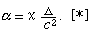If the true orbit is a circle, then the equation (1) becomes: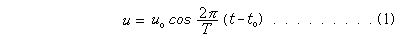If x is very small we find for the equation (2) the following approximate expression __________     1 Astronomische Kriterien für die Unabhängigkeit der Dortplanzungsgeschwindigkeit des Lichtes von der Bewegung der Lichtquelle, Astr. Nachr. 4670 (195, 265).     2 Zur Frage der Konstanz der Lichtgeschwindigkeit, Physik. Zeitschr. 14, 835). [*] Δ represents the distance from a double star to an observer. 396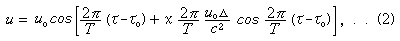where naturally u, uo, c, Δ, τ, and T must all be expressed in the same units (km. and sec.) The observed velocities will thus show a spurious excentricity, of the amount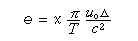This unites with the true excentricity and cannot be separated from it by observations.     Now it is easy to derive an upper limit for x. Take a well known star like β Aurigae. The observations give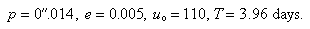As the largest values which are still compatible with the observations we can take p < 0".05,   or   Δ > 65 lightyears, e < 0.015,     We find then                                                                 x < 0.002.     Quite possibly other stars will give smaller values of x. The smallest values, of course, are found from the stars having the smallest parallaxes. Unfortunately the parallax of most spectroscopic doubles is still unknown, and it is thus impossible to give numerical values. We can however assume as certain that for the majority of these stars a value would be found which is still smaller than that given above.     Postscript. During the discussions at the meeting the remark was made (by Prof. Korteweg) that the star β Aurigae might have a true excentricity of such amount as exactly to cancel the spurious excentricity produced by the motion. This is, of course, entirely correct. If this true excentricity 1 were e = 0.90 we should find x = 0.12, [taking again p = 0".05 and using the same approximate formula as above, though this is not correct for such large excentricities]. Thus if we knew only this one star, we should have to adopt as upper limit for x this value 0.12. There are however a considerable number of stars with large values of uo, whose observed excentricity is very small or zero. Several of these certainly have very small parallaxes. It would evidently be absurd to assume that all of these possessed exactly that true excentricity and position of the periastron which would cancel the apparent excentricity for an observer on our earth. __________     1 For β Aurigae a large excentricity is particularly improbable on account of the presumably large dimensions of both components as compared with their mutual distance. Assistance was provided by the Interlibrary Loan Services at Mississippi State University's Mitchell Memorial Library and The Smithsonian Institution Libraries in obtaining a copy of the original "Proceedings" article. __________ Installed 01 Jun 2006. _________ Shade Tree Physics

Robert Fritzius
fritzius@bellsouth.net

Top